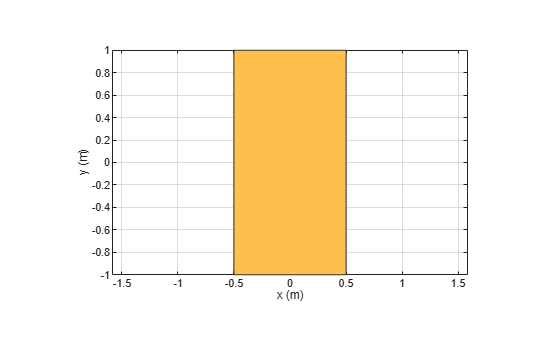# antenna.Rectangle

Create rectangle centered at origin on X-Y plane

## Description

Use the `antenna.Rectangle` object to create a rectangle centered at the origin and on the X-Y plane. You can use `antenna.Polygon` to create single-layer or multi-layered antennas using `pcbStack`.

## Creation

### Syntax

``rect = antenna.Rectangle``
``rect = antenna.Rectangle(Name,Value)``

### Description

example

````rect = antenna.Rectangle` creates a rectangle centered at the origin and on the X-Y plane.```

example

````rect = antenna.Rectangle(Name,Value)` sets properties using one or more name-value pair. For example, ```rectangle = antenna.Rectangle('Length',0.2)``` creates a rectangle of length 0.2 m. Enclose each property name in quotes.```

## Properties

expand all

Name of rectangle, specified a character vector.

Example: `'Name','Rect1'`

Example: `rectangle.Name = 'Rect1'`

Data Types: `char` | `string`

Cartesian coordinates of center of rectangle, specified a 2-element vector with each element measured in meters.

Example: `'Center',[0.006 0.006]`

Example: `rectangle.Center = [0.006 0.006]`

Data Types: `double`

Rectangle length, specified a scalar in meters.

Example: `'Length',2`

Example: `rectangle.Length = 2`

Data Types: `double`

Rectangle width, specified a scalar in meters.

Example: `'Width',4`

Example: `rectangle.Width = 4`

Data Types: `double`

Number of discretization points per side, specified a scalar.

Example: `'NumPoints',16`

Example: `rectangle.NumPoints = 16`

Data Types: `double`

## Object Functions

 `add` Boolean unite operation on two shapes `area` Calculate area of shape in square meters `subtract` Boolean subtraction operation on two shapes `intersect` Boolean intersection operation on two shapes `rotate` Rotate shape about axis and angle `rotateX` Rotate shape about X-axis and angle `rotateY` Rotate shape about Y-axis and angle `rotateZ` Rotate shape about Z-axis and angle `translate` Move shape to new location `show` Display antenna or array structure; display shape as filled patch `mesh` Mesh properties of metal or dielectric antenna or array structure

## Examples

collapse all

Create a rectangle shape using antenna.Rectangle and view it.

`r1 = antenna.Rectangle`
```r1 = Rectangle with properties: Name: 'myrectangle' Center: [0 0] Length: 1 Width: 2 NumPoints: 2 ```
`show(r1)`Create and view a rectangle with a length of 2 m and a width of 4 m.

```r2 = antenna.Rectangle('Length',2,'Width',4); show(r2) axis equal ```Rotate the rectangle.

```rotateZ(r2,45); show(r2) ```Create a rectangle with a length of 0.15 m, and a width of 0.15 m.

`r = antenna.Rectangle('Length',0.15,'Width',0.15);`

Create a second rectangle with a length of 0.05 m, and a width of 0.05 m. Set the center of the second rectangle at half the length of the first rectangle r.

`n = antenna.Rectangle('Center',[0.075,0],'Length',0.05,'Width',0.05);`

Create and view a notched rectangle by subtracting n from r.

```rn = r-n; show(rn)```Calculate the area of the notched rectangle.

`area(rn)`
```ans = 0.0212 ```